﻿ 基于有限元超收敛的三维节点型有限元后处理技术
 石油地球物理勘探2021, Vol. 56Issue (4): 882-890  DOI: 10.13810/j.cnki.issn.1000-7210.2021.04.0210文章快速检索 高级检索

### 引用本文TANG Jingtian, LIAO Taoshan, CHEN Huang, HUANG Xiangyu, ZHOU Feng, ZHANG Lincheng. Post-processing algorithm based on superconvergence for nodal 3D finite element modeling. Oil Geophysical Prospecting, 2021, 56(4): 882-890. DOI: 10.13810/j.cnki.issn.1000-7210.2021.04.021.### 作者简介陈煌, 湖南省长沙市麓山南路932号中南大学校本部地学楼, 410083。Email: csuchenhuang@csu.edu.cn

### 文章历史

① 中南大学有色金属成矿预测与地质环境监测教育部重点实验室, 湖南长沙 410083;
② 中南大学地球科学与信息物理学院, 湖南长沙 410083;
③ 中国能源建设集团湖南省电力设计院有限公司, 湖南长沙 410083;
④ 东华理工大学地球物理与测控技术学院, 江西南昌 330013;
⑤ 湖南城市学院信息与电子工程学院, 湖南益阳 413002

Post-processing algorithm based on superconvergence for nodal 3D finite element modeling
TANG Jingtian①② , LIAO Taoshan , CHEN Huang①②, HUANG Xiangyu , ZHOU Feng①④ , ZHANG Lincheng
① Key Laboratory of Metallogenic Prediction of Nonferrous Metals and Geological Environment Monitoring, Ministry of Education(Central South University), Changsha, Hunan 410083, China;
② School of Geosciences and Info-Physics, Central South University, Changsha, Hunan 410083, China;
③ China Energy Engineering Group Hunan Electric Power Design Institute Co., Ltd., Changsha, Hunan 410083, China;
④ School of Geophysics and Measurement-control Technology, East China University of Technology, Nanchang, Jiangxi 330013, China;
⑤ College of Information and Electronic Engineering, Hunan City University, Yiyang, Hunan 413002, China
Abstract: In the nodal finite element forward modeling of geophysical electromagnetic methods, the finite element solution of the main field needs to undergo numerical differentiation to derive the auxiliary field or the finite element calculation of potentials is necessary to yield the components of the electromagnetic field. To tackle the problem of low accuracy of traditional post-processing methods, we propose a method based on the superconvergent patch recovery (SPR) for the post-processing of nodal finite element forward modeling, which is applicable to the controlled-source electromagnetic method. First, on the basis of the curl-curl equation of the secondary electric field, the electric field (primary field) is solved with the Galerkin finite element method involving structured hexahedral grids and nodes. Then, given the superconvergence of the nodal finite element method, all adjacent elements at a certain node are used to form a patch. The electric field gradients are subjected to the least-squares surface fitting with Gauss points as sampling points on the patch so that the electric field gradients of nodes on the patch can be reco-vered. Finally, a high-precision magnetic field is achieved according to the recovered electric field gradients, and the high-precision apparent resistivity and phase responses are then obtained. The results show that the SPR-based post-processing algorithm can improve the accuracy of magnetic field components greatly and maintain good stability with slight increases in the memory and calculation time, compared with the conventional shape function differentiation (SFD), Lagrange interpolation (LI), and moving least-squares interpolation (MLSI).
Keywords: electromagnetic method    nodal finite ele-ment modeling    post-processing    superconvergent patch recovery
0 引言

Zienkiewicz等[15-20]发现有限元解的导数在某些特殊点上具有特别高的精度，并将这种有限元的超收敛性质用于渐进准确的Z-Z后验误差估计方法，系统地提出了SPR技术。由于SPR技术具有计算简洁、容易理解、效果显著及现有的有限元接口方便等优势，在计算流体力学、结构力学等领域已经得到广泛应用。在地球物理领域，Ren等基于该方法实现了直流电阻率法的三维非结构化网格自适应有限元正演。

1 SPR方法原理 1.1 CSEM满足的边值问题

 $\begin{array}{l} \nabla \times \nabla \times {{\boldsymbol{E}}^{\rm{s}}} - {\rm{i}}\omega {\mu _0}\left( {\sigma - {\rm{i}}\omega {{\boldsymbol{\varepsilon }}_0}} \right){{\boldsymbol{E}}^{\rm{s}}} + \nabla \left( {\nabla \cdot \sigma {{\boldsymbol{E}}^{\rm{s}}}} \right)\\ \;\;\;\;\;\;\;\; = {\rm{i}}\omega {\mu _0}\left( {\sigma - {\sigma _{\rm{p}}}} \right){{\boldsymbol{E}}^{\rm{p}}} \end{array}$ (1)

 ${{\boldsymbol{E}}^{\rm{s}}} = 0\;\;\;\;\;\;\;\;\; \in \Gamma$ (2)

1.2 有限元后处理算法

 ${\boldsymbol{H}} = \frac{1}{{{\rm{i}}\omega {\mu _0}}}(\nabla \times {\boldsymbol{E}})$ (3)

1.2.1 SPR后处理技术

SPR技术的原理简述如下：由围绕任一公共节点的所有相关单元组成一单元片，一般将单元片上高斯点的有限元解梯度作为采样对象，设近似多项式进行最小二乘曲面拟合，以恢复单元片上节点或其他特定点的梯度值，恢复的梯度值同样具有超收敛性。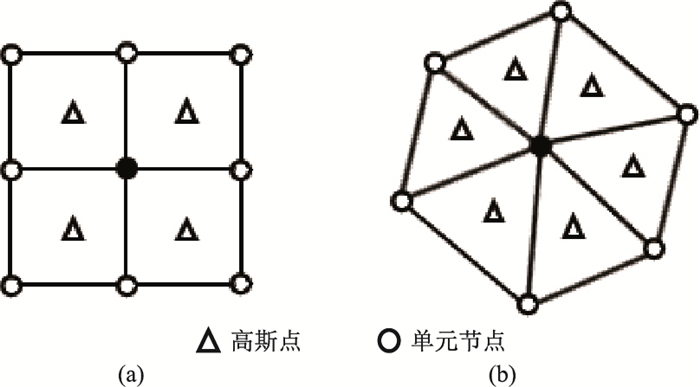图 1 二维线性插值单元片 (a)矩形单元片；(b)三角形单元片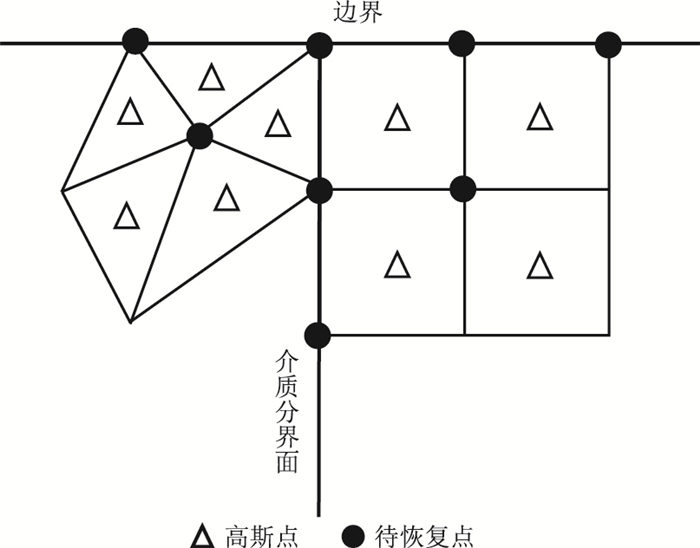图 2 介质边界及分界面上的二维单元片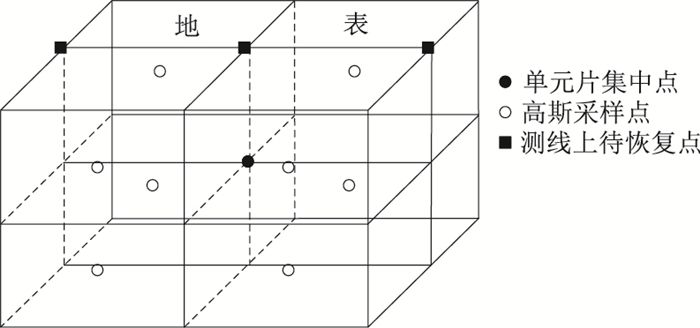图 3 六面体单元组成的单元片(三线性插值)示意图

 $\partial {{\boldsymbol{E}}^*} = {\boldsymbol{Pm}}$ (4)

 $\mathit{\Pi }({\boldsymbol{m}}) = \sum\limits_{i = 1}^n {{{\left( {\partial {{\boldsymbol{E}}_i} - \partial {\boldsymbol{E}}_i^*} \right)}^2}}$ (5)

 ${\boldsymbol{m}} = {{\boldsymbol{A}}^{ - 1}}{\boldsymbol{k}}$ (6)

1.2.2 单元形函数微分法(SFD)

 $\left\{ {\begin{array}{*{20}{l}} {x = {x_0} + \frac{a}{2}\xi }\\ {y = {y_0} + \frac{b}{2}\eta }\\ {z = {z_0} + \frac{c}{2}\gamma } \end{array}} \right.$ (7)

 ${N_i} = \frac{1}{8}\left( {1 + {\xi _i}\xi } \right)\left( {1 + {\eta _i}\eta } \right)\left( {1 + {\gamma _i}\gamma } \right)$ (8)

 ${E_p} = \sum\limits_{i = 1}^n {{N_i}} {E_{p, i}}\;\;\;\;p = x, y, z$ (9)

 $\left\{ {\begin{array}{*{20}{l}} {\frac{{\partial {E_p}}}{{\partial x}} = \frac{1}{{4a}}\sum\limits_{i = 1}^n {{\xi _i}} \left( {1 + {\eta _i}\eta } \right)\left( {1 + {\gamma _i}\gamma } \right){E_{p, i}}}\\ {\frac{{\partial {E_p}}}{{\partial y}} = \frac{1}{{4b}}\sum\limits_{i = 1}^n {{\eta _i}} \left( {1 + {\xi _i}\xi } \right)\left( {1 + {\gamma _i}\gamma } \right){E_{p, i}}}\\ {\frac{{\partial {E_p}}}{{\partial z}} = \frac{1}{{4c}}\sum\limits_{i = 1}^n {{\zeta _i}} \left( {1 + {\xi _i}\xi } \right)\left( {1 + {\eta _i}\eta } \right){E_{p, i}}}\\ {p = x, y, z} \end{array}} \right.$ (10)

1.2.3 拉格朗日插值(LI)法

LI法是已知节点的电场分量，令插值基函数lj(x)在点xj处取值为1，在其他点xi(ij)处取值为0。

 $\left\{ {\begin{array}{*{20}{l}} {{{\left. {\frac{{\partial {E_p}}}{{\partial x}}} \right|}_{{x_1}}} = \frac{{E_p^{{x_2}} - E_p^{{x_0}}}}{{2a}}}\\ {{{\left. {\frac{{\partial {E_p}}}{{\partial y}}} \right|}_{{y_1}}} = \frac{{E_p^{{y_2}} - E_{{p^0}}^{{y_0}}}}{{2b}}}\\ {{{\left. {\frac{{\partial {E_p}}}{{\partial z}}} \right|}_{{z_0}}} = \frac{{ - 11E_p^{{z_0}} + 18E_p^{{z_1}} - 9E_p^{{z_2}} + 2E_p^{{z_3}}}}{{6c}}} \end{array}} \right.$ (11)

1.2.4 移动最小二乘(MLSI)法

MLSI法被广泛应用于非结构化网格有限元正演的后处理。与SPR后处理技术不同，它的采样对象是节点上的电场分量，同时引入权函数，使距离中心点越远的点被赋予越小的权重，恢复局部域中任意位置的电场梯度

 $f = {\boldsymbol{U\alpha }}$ (12)

 $\omega (j) = {{\rm{e}}^{ - {\beta ^2}\left[ {{{\left( {{x_j}/{x_{\rm{m}}}} \right)}^2} + {{\left( {{y_j}/{y_{\rm{m}}}} \right)}^2} + {{\left( {{z_j}/{z_{\rm{m}}}} \right)}^2}} \right]}}$ (13)

 $F({\boldsymbol{\alpha }}) = \sum\limits_{j = 1}^N \omega (j){\left( {f_j^* - {f_j}} \right)^2}$ (14)

 ${\boldsymbol{\alpha }} = {{\boldsymbol{B}}^{ - 1}}{\boldsymbol{v}}$ (15)

 $\left\{ {\begin{array}{*{20}{l}} {\frac{{\partial f}}{{{\partial _{{x_K}}}}} = {\alpha _2}}\\ {\frac{{\partial f}}{{{\partial _{{y_K}}}}} = {\alpha _3}}\\ {\frac{{\partial f}}{{{\partial _{{z_K}}}}} = {\alpha _4}} \end{array}} \right.$ (16)

2 数值对比与分析 2.1 主程序验证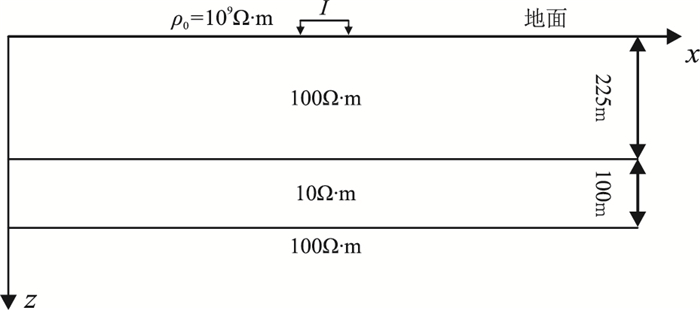图 4 一维低阻层状模型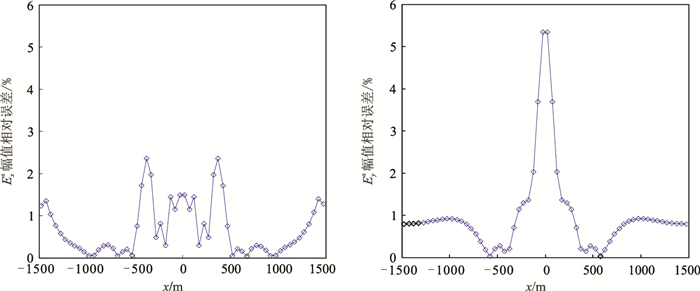图 5 二次电场分量128Hz时Exs(左)和Eys(右)的幅值相对误差
2.2 后处理算法数值对比分析

2.2.1 低阻层状异常体模型的二次场分析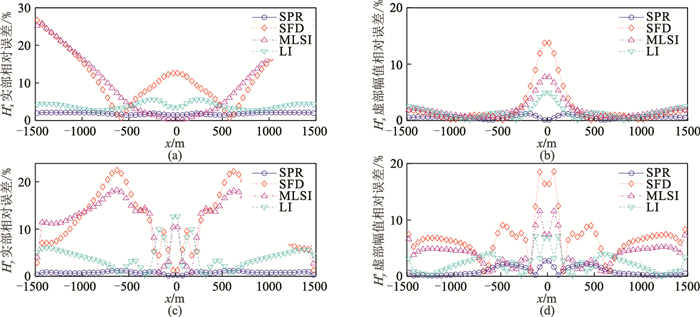图 6 低阻层状模型不同后处理算法二次磁场分量相对误差曲线(y=1500m，f=128Hz) (a)Hxs实部；(b)Hxs虚部；(c)Hys实部；(d)Hys虚部表 1 低阻层状模型四种后处理方法单测线相关参数统计

2.2.2 高阻层状异常体模型二次场分析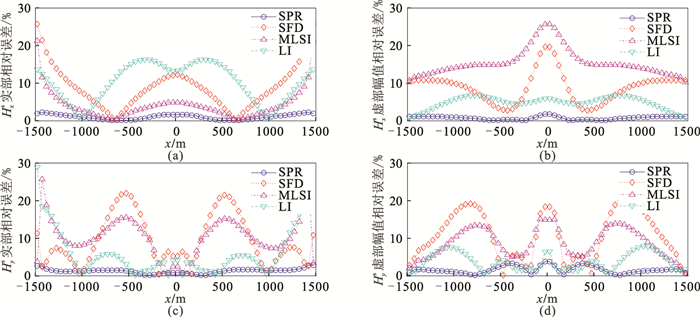图 7 高阻层状模型不同后处理算法二次磁场分量相对误差(y=1500m，f=256Hz) (a)Hxs实部；(b)Hxs虚部；(c)Hys实部；(d)Hys虚部表 2 高阻层状模型四种后处理方法二次磁场分量平均相对误差统计
2.2.3 均匀半空间模型的磁场总场分析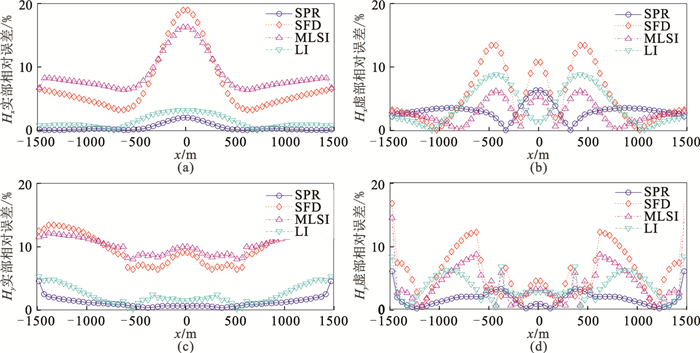图 8 高阻层状模型不同后处理算法磁场总场分量相对误差图(y=1500m，f=256Hz) (a)Hx实部；(b)Hx虚部；(c)Hy实部；(d)Hy虚部表 3 均匀半空间模型四种后处理方法磁场总场分量平均相对误差统计
2.2.4 三维高阻体模型的二次场分析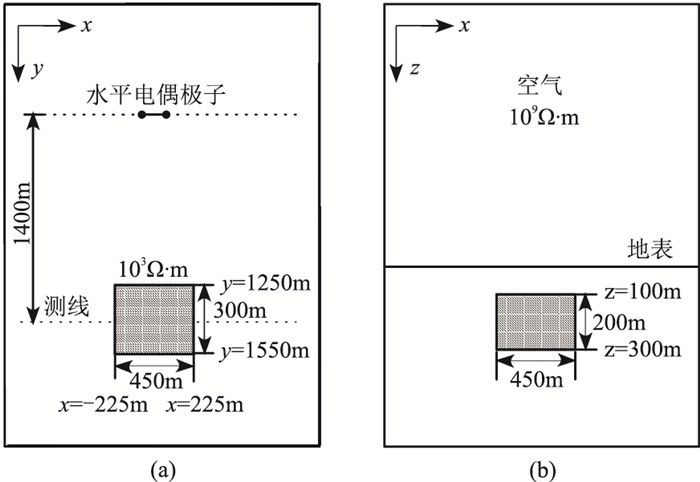图 9 三维高阻体模型 (a)俯视图；(b)侧视图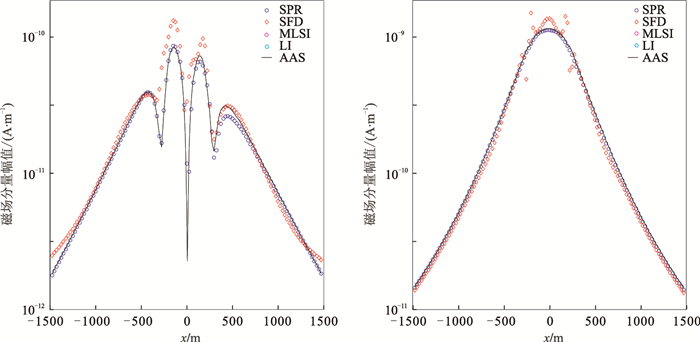图 10 三维高阻体模型不同后处理方法磁场二次场分量Hxs(左)和Hys(右)幅值曲线(y=1400m，f=256Hz)
3 结论

  吴桂桔, 胡祥云, 刘慧. CSAMT三维正演数值模拟研究进展[J]. 地球物理学进展, 2010, 25(5): 1795-1801. WU Guiju, HU Xiangyun, LIU Hui. Progress in CSAMT three-dimensional forward numerical simulation[J]. Progress in Geophysics, 2010, 25(5): 1795-1801.  陈乐寿, 王光锷. 大地电磁测深法[M]. 北京: 地质出版社, 1990.  Rodi W L. A technique for improving the accuracy of finite element solutions for magnetotelluric data[J]. Geophysical Journal International, 1976, 44(2): 483-506. DOI:10.1111/j.1365-246X.1976.tb03669.x  陈乐寿, 孙必俊. 有限元法在大地电磁场二维正演中的改进[J]. 石油地球物理勘探, 1982, 17(3): 69-76. CHEN Leshou, SUN Bijun. Improvement on the application of finite element method to 2-D forward solution of magnetotelluric field[J]. Oil Geophysical Prospecting, 1982, 17(3): 69-76.  陈小斌. 有限元直接迭代算法[J]. 物探化探计算技术, 1999, 21(2): 165-171. CHEN Xiaobin. Direct iterative algorithm of the finite element[J]. Computing Techniques for Geophy-sical and Geochemical Exploration, 1999, 21(2): 165-171. DOI:10.3969/j.issn.1001-1749.1999.02.010  陈小斌, 胡文宝. 有限元直接迭代算法及其在线源频率域电磁响应计算中的应用[J]. 地球物理学报, 2002, 45(1): 119-130. CHEN Xiaobin, HU Wenbao. Directly iterative finite element algorithm and its application in line current source electromagnetic response modeling[J]. Chinese Journal of Geophysics, 2002, 45(1): 119-130. DOI:10.3321/j.issn:0001-5733.2002.01.015  陈小斌, 张翔, 胡文宝. 有限元直接迭代算法在MT二维正演计算中的应用[J]. 石油地球物理勘探, 2000, 35(4): 487-496. CHEN Xiaobin, ZHANG Xiang, HU Wenbao. Application of finite-element direct iteration algorithm to MT 2-D forward computation[J]. Oil Geophysical Prospecting, 2000, 35(4): 487-496. DOI:10.3321/j.issn:1000-7210.2000.04.011  徐世浙. 地球物理中的有限单元法[M]. 北京: 科学出版社, 1994.  张继锋. 基于电场双旋度方程的三维可控源电磁法有限单元法数值模拟[D]. 湖南长沙: 中南大学, 2008.  张林成, 汤井田, 任政勇, 等. 基于二次场的可控源电磁法三维有限元-无限元数值模拟[J]. 地球物理学报, 2017, 60(9): 3655-3666. ZHANG Lincheng, TANG Jingtian, REN Zhengyong, et al. Forward modeling of 3D CSEM with the coupled finite-infinite element method based on the se-cond field[J]. Chinese Journal of Geophysics, 2017, 60(9): 3655-3666.  Badea E A, Everett M E, Newman G A, et al. Finite-element analysis of controlled-source electromagnetic induction using Coulomb-gauged potentials[J]. Geophysics, 2001, 66(3): 786-799. DOI:10.1190/1.1444968  Vladimir P, Jelena K, Josep D L P, et al. A parallel finite-element method for three-dimensional controlled-source electromagnetic forward modelling[J]. Geophysical Journal International, 2013, 56(2): 678-693.  蔡红柱, 熊彬, Michael Zhdanov. 电导率各向异性的海洋电磁三维有限单元法正演[J]. 地球物理学报, 2015, 58(6): 2839-2850. CAI Hongzhu, XIONG Bin, MICHAEL Zhdanov. Three-dimensional marine controlled-source electromagnetic modelling in anisotropic medium using finite element method[J]. Chinese Journal of Geophysics, 2015, 58(6): 2839-2850.  陈汉波, 李桐林, 熊彬, 等. 基于Coulomb规范势的电导率呈任意各向异性海洋可控源电磁三维非结构化有限元数值模拟[J]. 地球物理学报, 2017, 60(8): 3254-3263. CHEN Hanbo, LI Tonglin, XIONG Bin, et al. Finite-element modeling of 3D MCSEM in arbitrarily anisotropic medium using potentials on unstructured grids[J]. Chinese Journal of Geophysics, 2017, 60(8): 3254-3263.  Zienkiewicz O C, Cheung Y K. The Finite Element Method in Structural and Continuum Mechanics: Numerical Solution of Problems in Structural and Continuum Mechanics[M]. McGraw-Hill Publishing Company Limited, 1967.  Zienkiewicz O C, Zhu J Z. A simple error estimator and adaptive procedure for practical engineering analy-sis[J]. International Journal for Numerical Methods in Engineering, 1987, 24(2): 337-357. DOI:10.1002/nme.1620240206  Zienkiewicz O C, Zhu J Z. The superconvergent patch recovery and a posteriori error estimates, Part Ⅰ: The recovery technique[J]. International Journal for Numerical Methods in Engineering, 1992, 33(7): 1331-1364. DOI:10.1002/nme.1620330702  Zienkiewicz O C, Zhu J Z. The superconvergent patch recovery and a posteriori error estimates, Part Ⅱ: Error estimates and adaptivity[J]. International Journal for Numerical Methods in Engineering, 1992, 33(7): 1365-1382. DOI:10.1002/nme.1620330703  Zienkiewicz O C, Zhu J Z. The superconvergent patch recovery(SPR) and adaptive finite element refinement[J]. Computer Methods in Applied Mechanics & Engineering, 1992, 101(1): 207-224.  Zienkiewicz O C, Zhu J Z. Superconvergence and the superconvergent patch recovery[J]. Finite Elements in Analysis & Design, 1995, 19(1-2): 11-23.  Ren Z, Tang J. 3D direct current resistivity modeling with unstructured mesh by adaptive finite-element method[J]. Geophysics, 2010, 75(1): H7-H17. DOI:10.1190/1.3298690  Tabbara M, Blacker T, Belytschko T. Finite element derivative recovery by moving least square interpolants[J]. Computer Methods in Applied Mechanics & Engineering, 1994, 117(1-2): 211-223.